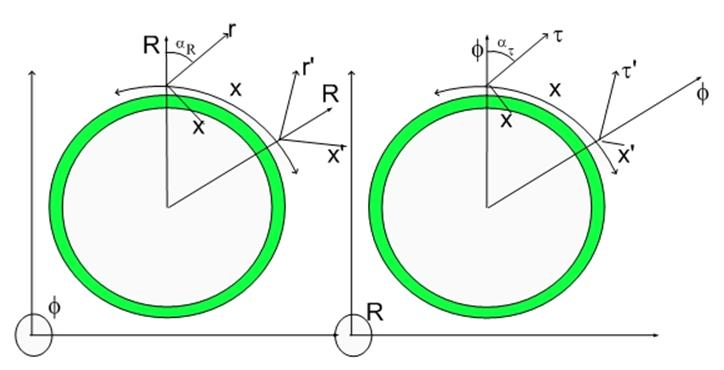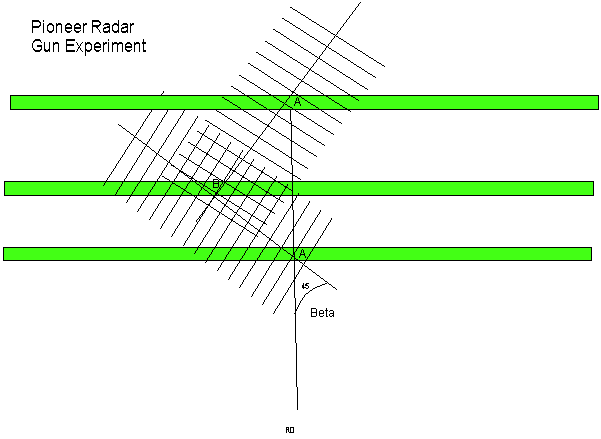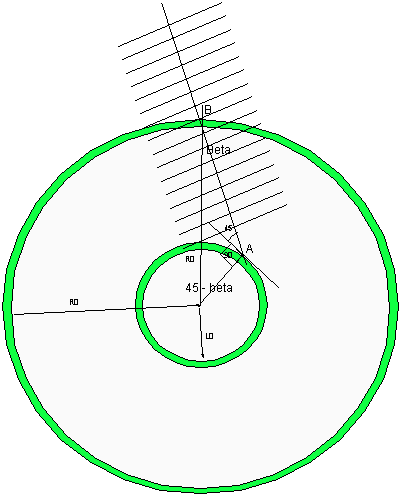# Darn Retarded Potentials and the Beginning of Times…:)Darn Retarded Potentials and the Beginning of Times…:)

In the past few postings I reached a conclusion which I cannot refute within the logical framework of my theory.

The conclusion I reached is that the actual speed of light is Sqrt(2) times the measured speed of light.:)

Or that light is faster than itself (or what we perceive as being its speed..:)

Up to now my mind has been living with a conundrum… a paradox. The equations of motion, the Unification Equation is derived from a simple principle that dilators never do any work as they move. There are two kinds of motion. Radial and lateral, where lateral means within our 3D Universe (a lightspeed expanding Hypersphere).

The equations of motion for lateral movement required the mapping of inertia to the footprint (on specific phases of the spinning motion) of the dilator on our 3D Hypersurface.

There was no equivalent inertia mapping for radial motion. That never caused a problem because we never had time to fully develop the initial Cosmogenesis process.

When I worked on calculating the age of the Universe from the Pioneer Anomaly, I had to struggle with something obvious that I had overlooked for a few years. The problem is the following: If I am surfing, can I make a wave on the surface of the wave and see it propagate? The solution to the old problem of waves in a wave eluded me for many years.

For a dilator to move always without doing any work, the fastest it can ever travel is surfing the dilaton wave generated by other dilators. The larger the dilaton field the highest the acceleration but the highest speed is always c (the observed speed of light).

The paradox is that lateral motion requires inertia while the initial setting in motion of the whole Universe never seemed to require inertia…:) I considered the motion of the dilator (which is a coherence thus not unlike a wave) to be traveling at its natural speed. That was satisfactory explanation until I faced the Pioneer Anomaly problem…;)

The dependence of the observed velocity as a function of observed Epoch (beta angle) was necessary to keep the dilator frequency unchanged. The Hubble related red-shifting had to do only with the geometric projection of the waves into the lightspeed expanding Hypersphere (our Universe).

For that to happen the actual speed of light had to be higher than the observed speed of light by a factor of sqrt(2).

This means that dilators always have the same amount of Inertia for both lateral and radial motion.

Retarded Potential

I remember when I read Landau and Lifshits for the first time about Retarded Potentials. We all know that the Sun is eight light minutes from us, that is, we are always seeing what was happening to the Sun eight minutes ago. The field propagation travels at the speed of light for both Gravity and Electromagnetism. This is convenient since both are described as dilaton field modulations in my theory.

First let's consider that the 3D Universe is already in motion. Here we have the Universe already in motion and all interaction between particles in different positions (relatively close) travel at 45 degrees and thus at c.
This is consistent with my Quantum Lagrangian Principle where dilators always surf the local metric dilaton field. The fastest this dilaton field can drag a dilator with its own local velocity or the observed speed of light (consistent with observation and relativity).Figure 1 displays the geometry for peering or interacting with the near past. From the past blog, we established that the actual speed of light is sqr(2) times the observed speed of light.

I cannot create a perfect explanation. It would take time. On the other hand, it should be self-evident that this is the only solution to the beginning of times..:) just by looking at the following figure:Figure 2. Interacting with the past.  One always interact with the past through Retarded Potenials...:)

It turns out that the same picture that describes how do we currently look into the past can provide insight on the first moment of this Universe.

Let's consider that in the first instant of this Universe there was two layers of Universe (Biverse)…:) strongly interacting (Gravitation, radiation) etc.

If you consider that the Hypersphere to be a point, tangential motion is the same as radial motion. The only angle which allows dilators to travel at the same tangential speed as the radial speed is 45 degrees interaction.

The symmetry of the problem forces that the radial acceleration to be equal to the tangential acceleration (think about this as basically a point in the 4D spatial manifold).

This means that if one consider that radial and tangential accelerations or speed should yield the same hyperspherical topology at the end if one starts from a point, them the interaction has to take place at 45 degrees and the equilibrium condition is all dilators traveling at the observed speed of light tangentially and radially at the beginning of times.

That acceleration took no time as motion is just the surfing upon the dilaton field. Given a strong enough dilaton field and everything will travel at the speed of light…:)

Is there a need for an initial Biverse? No it was just drawn to show the interaction symmetry. When one interacts with another the other is always in the past.  If this interaction is repulsive (as being blown away by gamma radiation, neutrino flows) the originator of the neutrino and the receiver of the momentum are always in different epochs (different radius) of the Universe.

When the Universe is a point, interaction that produces tangential motion also produces radial motion. From a point tangential motion is the same as radial motion.

If you consider that the Four Dimensional Big Bang (created by the dilaton field) creates equally distributed radial and tangential motions, then the speed of the shock wave Hypersphere has to be equal to the dilaton field speed divided by sqrt(2).

From that point on, all potentials were called Retarded Potentials…:)

One more point, in the past I proposed a bipolar (TEM01) initial metric deformation as the format of the initial metric deformation:

This would lead to a bipolar hyperspherical Universe, that is, matter and antimatter would exist in different halves of the Universe with an empty boundary due to mutual assured annihilation of matter and antimatter...:)

It just happens that it is impossible to see anything farther than 45 degrees away from you, let alone 180 degrees from you.  By this reasoning we could never see any sign of the original annihilation unless we lived in that neighborhood.

Since then, I started thinking about TEM00. Well, that is also a possibility, in this case the Universe would be perfectly symmetric (not perfectly homogeneous).
In my Cosmogenesis reasoning, I considered that the creation of the Universe occurred together with a dimensional unfolding, that is, metric fluctuations not only changed the local spatial density but also the dimensionality of the space.  This reasoning was based on entropy considerations, after all the Universe where we live is a long lived fluctuation, certainly reversible but long lived due to entropy.

Not unlike finding your other half... It is possible but might take the age of the Universe..:)

Cheers,

MP

PS- I found a more succinct argument.  Consider my Quantum Lagrangian Principle which I used to unify all forces and  to calculate form first principles Cosmological Constants (G, epsilon0, mu0 etc).  I explained that the dilators should always surf (dilate in phase with the surrounding dilaton field).  To dilate in phase, each dilator has to move at each de Broglie step of the hyperspherical Universe expansion.
This surfing takes place on both 3D and 4D, that is the dilator is always in phase with the dilaton field which is a metric deformation in a 4D spatial manifold. The only way that the dilator will be in phase with both the 3D projection of the dilaton field  and with the 4D dilaton field itself is if initially accelerated to the speed of light both tangentially and radially.  Starting from a point, the Universe was formed as a flash of lighspeed traveling dilators traveling within a lightspeed expanding hyperspherical shockwave.

This is kind of succinct.  Please let me know if you can articulate it better.  I will be happy to post it and acknowledge your contribution to the discussion..:) Cheers
Currently unrated

### New Comment

required

required (not published)

optional

required